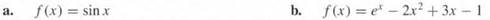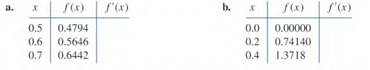# Use the forward-difference formulas and backward-difference formulas to determine each missing…

The data in Exercise 1 were taken from the following functions. Compute the actual errors in Exercise 1, and find error bounds using the error formulas.Exercise 1

Use the forward-difference formulas and backward-difference formulas to determine each missing entry in the following tables,